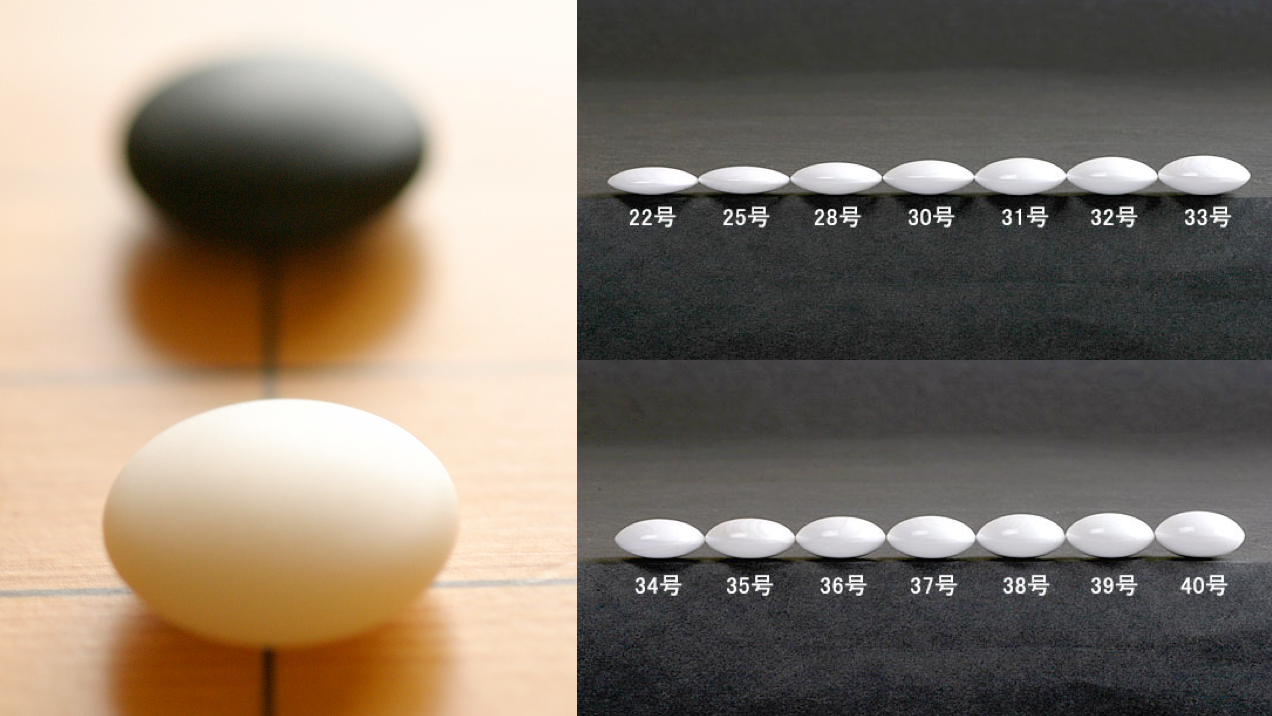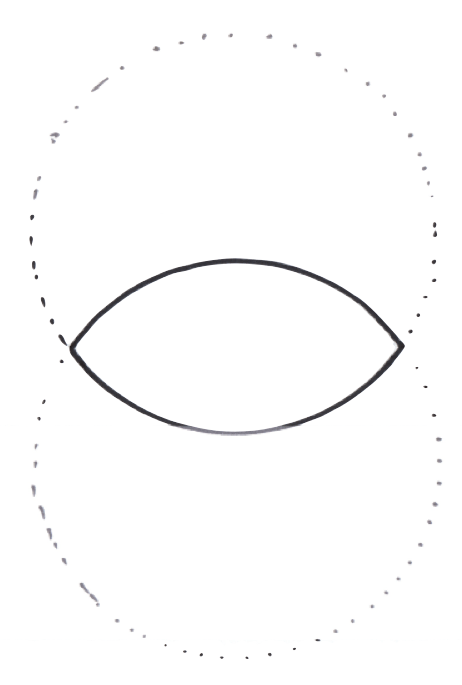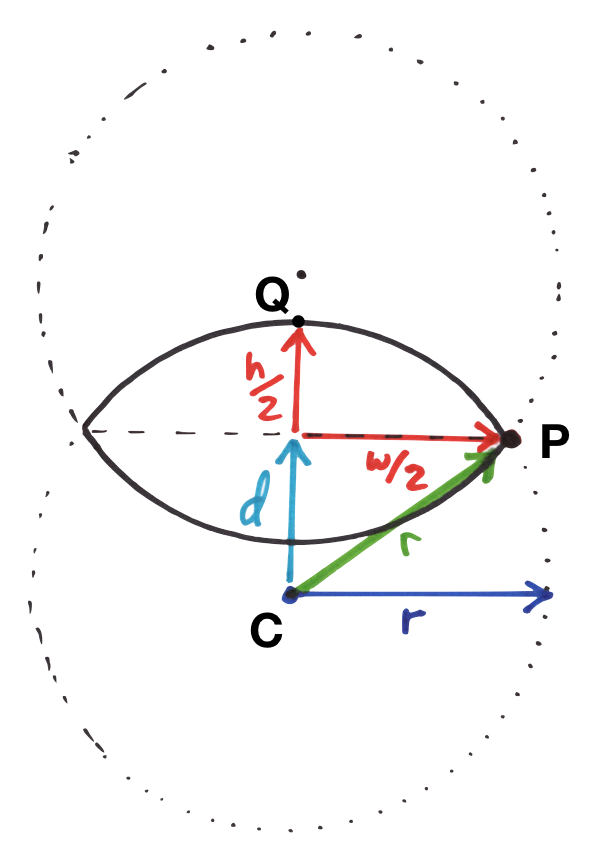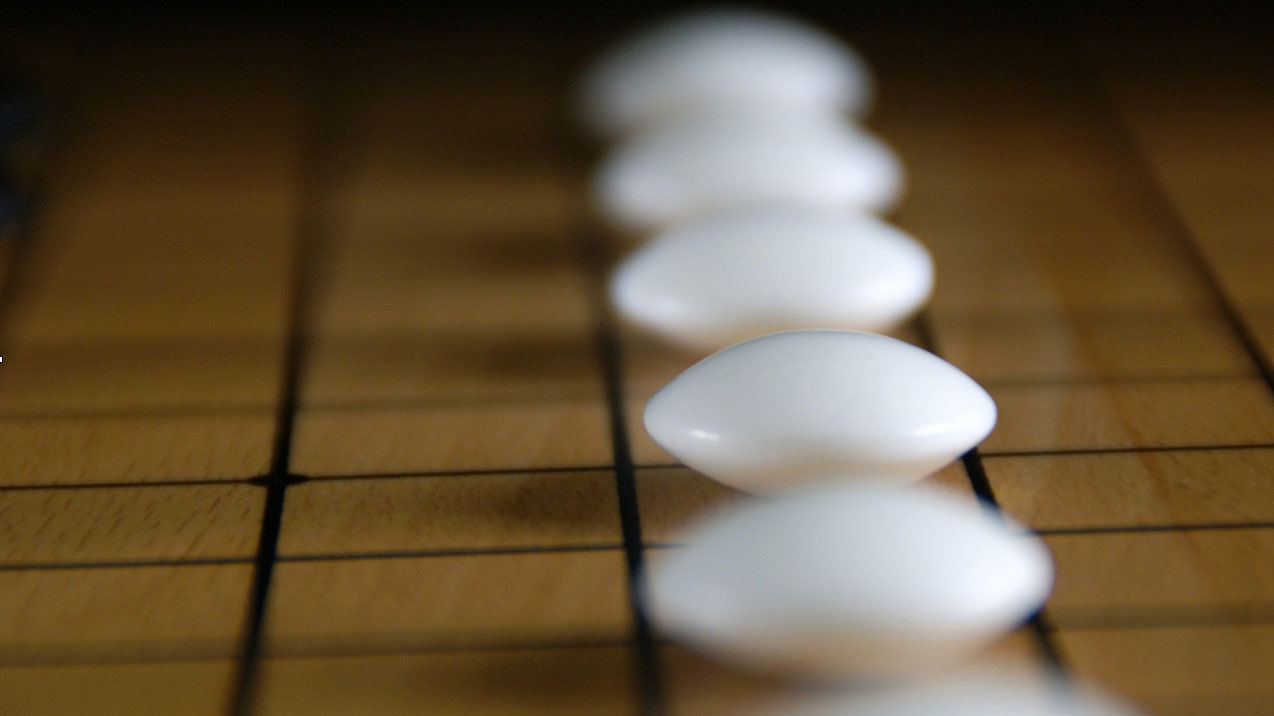# Shape of The Go Stone

## Introduction

Hi, I’m Glenn Fiedler. Welcome to Virtual Go, my project to create a physically accurate computer simulation of a Go board and stones.

If you play Go, you know that a biconvex go stone has an interesting wobble when it’s placed on the board. This wobble is a direct consequence of its unique shape.

I’d like to reproduce this wobble in Virtual Go, so let’s to spend some time studying go stone’s shape, so we can capture this wobble and simulate it on a computer :)

## Slate And Shell

In Japan, Go stones are traditionally made out of slate and clam shell.Clam shell stones come in several grades of quality. The highest being yuki or “snow” grade with fine, regularly spaced lines.

Go stones also come in different sizes. In general, the thicker the stone, the more expensive it is, as only a small portion of the clam shell is suitable for making them.At first glance the go stone looks like an ellipse, but side-on you can see this is not the case. This shape is called a biconvex solid. I find this shape interesting because it is the intersection of two spheres.

We can study this shape by looking at the intersection of two circles:I quickly noticed that by varying the radius of the circles and the distance between their centers, I could generate go stones of different sizes.

But when creating a go stone I don’t really want it to be parameterized this way.

Instead I’d like to say, “Hey, I would like a stone of this width and height” and have a function that calculates the radius of the circles and how far apart they should be to generate that stone.

To write this function we first need to do some math:First notice that the point Q lies on the generating circle, so the line CQ has length r:

todo: obviously I have a bunch of work to get the latex equations ported across to Hugo. I’m researching different options…

[latex size=“2”]d + h/2 = r[/latex] [latex size=“2”]d = r - h/2[/latex]

The point P is also on the generating circle so the green line CP has length r as well. Using Pythagoras theorem and substituting for d:

[latex size=“2”]r^2 = d^2 + (w/2)^2[/latex] [latex size=“2”]r^2 = ( r - h/2 )^2 + (w/2)^2[/latex] [latex size=“2”]r^2 = ( h^2/4 - hr + r^2 ) + w^2/4[/latex] [latex size=“2”]r^2 = h^2/4 - hr + r^2 + w^2/4[/latex] [latex size=“2”]0 = h^2/4 - hr + 0 + w^2/4[/latex] [latex size=“2”]hr = h^2/4 + w^2/4[/latex] [latex size=“2”]r = ( h^2 + w^2 ) / 4h[/latex]

Which gives us everything we need to write the function:

    void calculateBiconvex( float w,
float h,
float & r,
float & d )
{
r = ( w*w + h*h ) / ( 4*h );
d = r - h/2;
}


Now we can mathematically define a go stone parameterized by its width and height. There is just one problem: the edge is very sharp!

To make our stone aesthetically pleasing, lets round the edge with a bevel. Otherwise, you might cut yourself virtually when you play with it:Let’s parameterize the bevel by its height b:In three dimensions the bevel is actually a torus (donut) around the edge of the go stone. We need to calculate the major and minor radii r1 and r2 of the torus as a function of b and the dimensions of the go stone:The key to solving this is to realize that if the go stone and the bevel are to match perfectly then the tangent of the two circles must be equal at the point P.

Update: A few years later and it occurs to me that it would be even more beautiful if the second derivative matched at this intersection as well. Is this possible in general, or must the generating spheres become to ellipses in order to make this happen? I suspect this is the case. Mathematicians who play Go, let me know your thoughts.If the tangent is equal then the normal must be equal as well. This means that the center of the bevel circle lies at the intersection of the line CP and the x axis.

We already know C so if we can find the point P then we can find this intersection point. Once we know the intersection point we can find r1 and r2.

Since P is at the start of the bevel:

[latex size=“2”]P_y = b/2[/latex]

Because P lies on the biconvex circle with center C and radius r we can use the equation of the circle to find x as a function of y:

[latex size=“2”]x^2 + y^2 = r^2[/latex] [latex size=“2”]x = \sqrt{ r^2 - y^2 }[/latex]

We need y relative to the circle center C, not in go stone coordinates, so we add d and substitute y’ for y:

[latex size=“2”]y’ = b/2 + d[/latex] [latex size=“2”]P_x = \sqrt{ r^2 - ( b/2 + d )^2 }[/latex]

We can now find r1 by similar triangles:

[latex size=“2”]r_1/P_x = d / ( d + b/2 )[/latex] [latex size=“2”]r_1 = P_x d / ( d + b/2 )[/latex]

and q by Pythagoras theorem:

[latex size=“2”]q^2 = d^2 + r_1^2[/latex] [latex size=“2”]q = \sqrt{ d^2 + r_1^2 }[/latex]

Because line CP has length r and substituting for q:

[latex size=“2”]q + r_2 = r[/latex] [latex size=“2”]r_2 = r - q[/latex] [latex size=“2”]r_2 = r - \sqrt{ d^2 + r_1^2 }[/latex]

Now we have everything we need to write the function:

    void calculate_bevel( float r, float d, float b,
float & r1, float & r2 )
{
const float y = b/2 + d;
const float px = sqrt( r*r - y*y );
r1 = px * d / ( d + b/2 );
r2 = r - sqrt( d*d + r1*r1 );
}


Now we can calculate the bevel torus to round off any go stone we create as the intersection of two spheres.

NEXT ARTICLE: Tessellating The Go Stone

Glenn Fiedler is the founder and CEO of Network Next.
Network Next is fixing the internet for games by creating a marketplace for premium network transit.